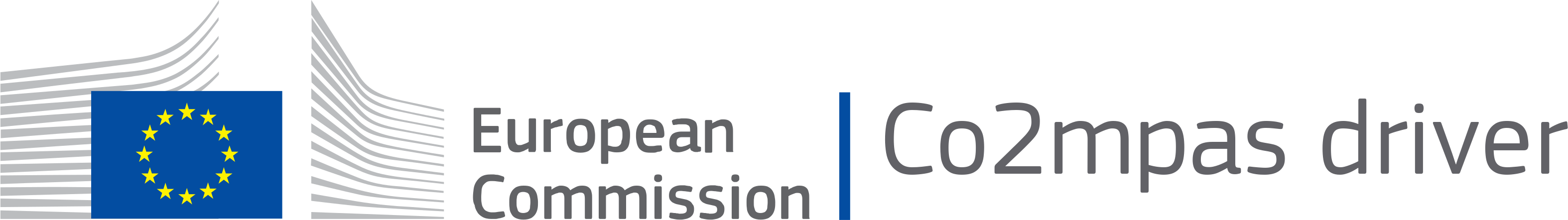A lightweight microsimulation free-flow acceleration model(MFC) or co2mpas_driver is a model that is able to capture the vehicle acceleration dynamics accurately and consistently

## Project descriptionversions:documentation:

sources:
keywords:

automotive, car, cars, driver, MCF, driving, simulation, simulator, standard, vehicle, vehicles, driver characterisation, driver profile, free-flow acceleration model, acceleration dynamics, driver behaviour, fuel/energy consumption

short name:

co2mpas_driver

live-demo:Licensed under the EUPL, Version 1.2 or â€“ as soon they will be approved by the European Commission â€“ subsequent versions of the EUPL (the “Licence”); You may not use this work except in compliance with the Licence. You may obtain a copy of the Licence at:Unless required by applicable law or agreed to in writing, software distributed under the Licence is distributed on an “AS IS” basis, WITHOUT WARRANTIES OR CONDITIONS OF ANY KIND, either express or implied. See the Licence for the specific language governing permissions and limitations under the Licence.

## Introduction

Co2mpas_driver is a library used to implement the microsimulation free-flow acceleration model (MFC). The MFC is able to accurately and consistently capture the acceleration dynamics of road vehicles using a lightweight and parsimonious approach. The model has been developed to be integrated in traffic simulation environments to enhance the realism of vehicles movements, to explicitly take into account driver behaviour during the vehicle acceleration phases, and to improve the estimation of fuel/energy consumptions and emissions, without significantly increasing their computational complexity. The proposed model is valid for both internal combustion engine and battery electric vehicles. The MFC has been developed by the Joint Research Centre of the European Commission in the framework of the Proof of Concept programme 2018/2019. For more details on the model please refer to Makridis et al. (2019) and He et al. (2020).

## Installation

### Prerequisites

Python-3.6+ is required and Python-3.7+ recommended.

• either with git, by giving this command to the terminal:

git clone https://github.com/JRCSTU/co2mpas_driver --depth=1

### Install

From within the project directory, run one of these commands to install it:

• for standard python, installing with pip is enough:

pip install -e .[path_to_co2mpas_driver]

or from @master branch:

pip install git+https://github.com/JRCSTU/co2mpas_driver.git@master

### Uninstall

To uninstall the package, run the following command from the project directory:

pip uninstall co2mpas_driver

## Usage

In this example we will use co2mpas_driver model in order to extract the drivers acceleration behavior as approaching the target speed.

### Setup

• First, set up python, numpy, matplotlib.

Set up python environment: numpy for numerical routines, and matplotlib for plotting

>>> import numpy as np
>>> import matplotlib.pyplot as plt

• co2mpas_driver must be imported as a dispatcher (dsp). The dsp contains functions to process vehicle data and run the com2pas_driver model. Also is necessary to import schedula for selecting and executing functions from the co2mpas_driver. For more information on how to use schedula: https://pypi.org/project/schedula/

>>> from co2mpas_driver import dsp
>>> import schedula as sh


• Load vehicle data for a specific vehicle from vehicles database

>>> db_path = 'EuroSegmentCar.csv'

• Load user input parameters from an excel file

>>> input_path = 'sample.xlsx'

• Sample time series

>>> sim_step = 0.1 #The simulation step in seconds
>>> duration = 100 #Duration of the simulation in seconds
>>> times = np.arange(0, duration + sim_step, sim_step)


>>> inputs = {
'vehicle_id': 35135,  # A sample car id from the database
'inputs': {'gear_shifting_style': 0.7, #The gear shifting style as
described in the TRR paper
'starting_speed': 0,
'desired_velocity': 40,
'driver_style': 1},  # gear shifting can take value
# from 0(timid driver) to 1(aggressive driver)
'time_series': {'times': times}
}


### Dispatcher

• Dispatcher will select and execute the proper functions for the given inputs and the requested outputs

>>> core = dsp(dict(db_path=db_path, input_path=input_path, inputs=inputs),
outputs=['outputs'], shrink=True)

• Plot workflow of the core model from the dispatcher

>>> core.plot()


This will plot the workflow of the core model on an internet browser (see below). You can click all the rectangular boxes to see in detail the sub-models like load, model, write and plot.Merged vehicle data for the vehicle_id used above• Load outputs of dispatcher and select the chosen dictionary key (outputs) from the given dictionary.

>>> outputs = sh.selector(['outputs'], sh.selector(['outputs'], core))

• Select the desired output

>>> output = sh.selector(['Curves', 'poly_spline', 'Start', 'Stop', 'gs',
'discrete_acceleration_curves', 'velocities',
'accelerations', 'transmission'], outputs['outputs'])


The final acceleration curves, the engine acceleration potential curves (poly_spline), start, stop, gear shift, discrete acceleration curves, velocities, accelerations and transmission, before calculating the resistances and the limitation due to max possible acceleration (friction).

>>> curves, poly_spline, start, stop, gs, discrete_acceleration_curves,
velocities, accelerations, transmission =
output['Curves'], output['poly_spline'], output['Start'], output['Stop'], output['gs'],
output['discrete_acceleration_curves'], output['velocities'],
output['accelerations'], output['transmission']


### Plot

>>> plt.figure('Time-Speed')
>>> plt.plot(times, velocities)
>>> plt.grid()
>>> plt.figure('Speed-Acceleration')
>>> plt.plot(velocities, accelerations)
>>> plt.grid()
>>> plt.figure('Acceleration-Time')
>>> plt.plot(times, accelerations)
>>> plt.grid()

>>> plt.figure('Speed-Acceleration')
>>> for curve in discrete_acceleration_curves:
sp_bins = list(curve['x'])
acceleration = list(curve['y'])
plt.plot(sp_bins, acceleration, 'k')
>>> plt.show()


### ResultsFigure 1. Speed(m/s) versus time(s) graph over the desired speed range.

Acceleration(m/s*2) versus speed(m/s) graphFigure 2. Acceleration per gear, the gear-shifting points and final acceleration potential of our selected

vehicle over the desired speed range

Acceleration(m/s*2) versus speed graph(m/s)Figure 3. The final acceleration potential of our selected vehicle over the desired speed range.

## Project details

This version1.3.41.3.31.3.11.3.01.2.01.1.01.0.21.0.0

Uploaded source